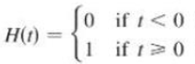Chapter 1.3, Problem 59E### Single Variable Calculus: Early Tr...

8th Edition
James Stewart
ISBN: 9781305270343

#### Solutions

Chapter
Section### Single Variable Calculus: Early Tr...

8th Edition
James Stewart
ISBN: 9781305270343
Textbook Problem

# The Heaviside function H is defined byIt is used in the study of electric circuits to represent the sudden surge of electric current, or voltage, when a switch is instantaneously turned on.(a) Sketch the graph of the Heaviside function.(b) Sketch the graph of the voltage V(t) in a circuit if the switch is turned on at time t = 0 and 120 volts are applied instantaneously to the circuit. Write a formula for V(t) in terms of H(t).(c) Sketch the graph or the voltage V(t) in a circuit if the switch is turned on at time t = 5 seconds and 240 volts are applied instantaneously to the circuit. Write a formula for V(t) in terms of H(t). (Note that starting at t = 5 corresponds to a translation.)

(a)

To determine

To sketch: The graph of the Heaviside function.

Explanation

The Heaviside function is, H(t)={0      if t<01

(b)

To determine

To sketch: The graph of V(t) in a circuit if the switch is turned on at time t = 0 and 120 volts are applied to the circuit; write the formula for V(t) in terms of H(t).

(c)

To determine

To sketch: The graph of the voltage in a circuit if the switch is turned on at t = 5 seconds and 240 volts are applied to the circuit; write the formula for V(t) in terms of H(t).

### Still sussing out bartleby?

Check out a sample textbook solution.

See a sample solution

#### The Solution to Your Study Problems

Bartleby provides explanations to thousands of textbook problems written by our experts, many with advanced degrees!

Get Started

#### Find more solutions based on key concepts